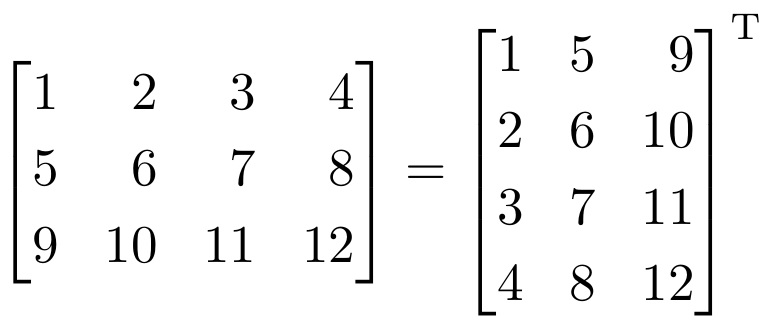# Matrix Transpose of a given Matrix of any dimension in Python3?

In this Python tutorial, we will learn how the matrix transpose of a matrix in Python.

## MATRIX TRANSPOSE in Python

Matrix transpose is an operation that can be clear from the following points:

• There’s a 3X3 matrix suppose named A.
• The diagonal elements of A (00, 11, 22) acts as a mirror
• The lower triangle elements are swapped into their respective image position.
• This same for the upper triangle elements too.
• In short, the linear arrangement of 90 degrees clockwise rotated rows of the matrix.

Below picture is an example of Transpose:MATRIX TRANSPOSE

Now, let’s take a look at the code snippet.

## PROGRAM: Matrix Transpose of a given Matrix of any dimension in Python

```print("Dimension of the Matrix:")
r=int(input("Rows="))
c=int(input("Columns="))
a=[]
print("Input the elements in",r,"x",c,":")
for i in range(r):
l=list(map(int,input().split(",")))
a.append(l)
#swapping
for i in range(r):
for j in range(c):
t=a[i][j]
a[i][j]=a[j][i]
a[j][i]=t
print("Transpose of Matrix:")
for i in range(r):
for j in range(c):
print(a[i][j],end=" ")
print()```

OUTPUT 1:

```Dimension of the Matrix:
Rows=3
Columns=3
Input the elements in 3 x 3 :
1,2,3
4,5,6
7,8,9
Transpose of Matrix:
1 2 3
4 5 6
7 8 9```

OUTPUT 2:

```Dimension of the Matrix:
Rows=3
Columns=5
Input the elements in 3 x 5 :
7,5,4,6,2
1,2,3,4,5
6,7,5,4,9
Transpose of Matrix:
7 1 6
5 2 7
4 3 5
6 4 4
2 5 9```

Also Read:

### One response to “Matrix Transpose of a given Matrix of any dimension in Python3?”

1.Saruque Ahamed Mollick says:

def transpose_matrix(matrix):
return zip(*matrix)